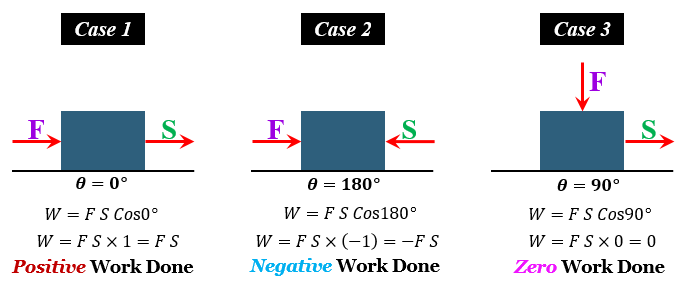# e and the displacement caused due to the force is 180°, then the displacement is in the opposite direction of the applied force.

Work is defined as the energy transferred to or from an object via the application of force causing the movement or displacement of an object.

It is commonly represented as the product of force in the direction of the displacement and the magnitude of this displacement.

Mathematically, it is given as-

$W=Fdcos\theta$

Where,

work done by the force.

F = force.

d = displacement caused by the force.

θ = angle between the force and the displacement.

(a.) If the angle between the applied force and the displacement caused due to the force is 0°, then the displacement is in the same direction of the applied force.

In this case, work done by the force would be "positive" because the angle, θ = 0°, and value of  Cos 0° = 1.

Example- When a man drops an object from his hands, the force of gravitation acts on the object in the downward direction. The displacement is also in the downward direction making an angle of  with respect to the force of gravity. Here, the work done by the gravitational force on the body is positive.

(b.) If the angle between the applied force and the displacement caused due to the force is 180°, then the displacement is in the opposite direction of the applied force.

In this case, work done by the force would be "negative" because the angle, θ = 180°, and value of  Cos 180° = -1.

Example-  When a man lifts an object from the ground, the force of gravity acts in the downward direction. But, the displacement of the body is in the upward direction making an angle of 18 with respect to the force of gravity. Here, the work done by the gravitational force on the body is negative.

⇒There is also a case in which the angle between the applied force and the displacement caused due to the force is 90°, then the displacement is perpendicular to the direction of the force of the applied force.

In this case, work done by the force would be "zero" because the angle, θ = 90°, and value of  Cos 90° = 0.

Example- A man lifting a mass on his head moving at an angle of 90° with respect to the force of gravity. Here, the work done by gravity on the object is zero.Updated on: 10-Oct-2022

38 Views